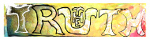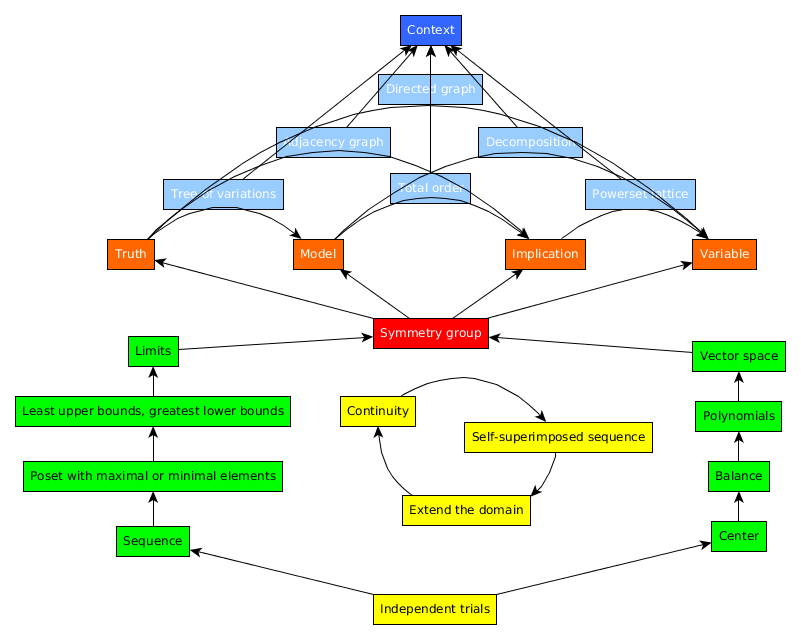发现 ms@ms.lt +370 607 27 665 My work is in the Public Domain for all to share freely. Software Submitted to the Third international conference of the Italian Network for the Philosophy of Mathematics, May 25-27, 2018, Mussomeli – Caltanissetta, Sicily, Italy. Identifying and Classifying Cognitively Natural Mathematical Constructs I wish to find a vantage point from which it would be possible to make sense of all of mathematics. What mathematical concepts, disciplines and truths can arise? and how and why? My strategy is to study how our mathematical activity is dictated by our limited imagination. Specifically, I have observed that each time we solve a mathematical challenge, our minds apply some classic mathematical construct. We can thus distinguish the explicit mathematics, on paper, which is often highly contrived, and the implicit mathematics, in our mind, which is inherently, cognitively natural. I survey these natural constructs and suggest how we can clarify them and systematize them. I start with an illustrative example. George Polya (1945), in his book, "How to Solve It", considers Euclid's problem of how to construct an equilateral triangle. If we are given one side AB, how do we construct the other two? The solution is a recurring idea which Polya calls the "pattern of two loci". We think of there being two separate conditions. One side must extend a length AB from the point A. Another side must extend a length AB from the point B. We thus draw two circles of radius AB centered at A and B. The points where the two circles intersect are those where we can draw a third point C which satisfies both conditions so that our triangle is equilateral. I realized that our minds solve this problem by imagining a powerset lattice of conditions. Circle A is one condition, circle B is another condition, and the intersection of A and B satisifies the union of these two conditions. Our minds have thus solved the surface problem (constructing an equilateral triangle) by considering a simpler, deeper structure (a lattice of conditions). This brings to mind linguist Noah Chomsky's work in syntax and architect Christopher Alexander's work on pattern languages. I collected such problem solving patterns discussed in Paul Zeitz's (2006) book "The Art and Craft of Problem Solving" and other sources. Each distinct pattern makes use of a structure which is familiar to mathematicians and yet is not explicit but mental. My approach is reminiscent of Lakoff and Nunez (2003), "Where Mathematics Comes From", except that they think of math as embodied and concrete, developing from what we know, whereas I think of math as developing from our abstract life, engaging what we don't know. I present to you 24 patterns for which there seems to be evidence. A) Algebra The crux of the problem may be to imagine: A0) The proper center or origin. A1) Parity or balance, as when modifying an equation with terms that add to zero or multiply to one, or in distinguishing a set and its complement, what is and is not. A2) A multiset, whose elements we can logically manipulate, as we do the roots of polynomials. A3) A list, for example, canonical basis elements for a vector subspace. B) Analysis In order to solve a problem, we often must imagine: B0) An ever growing sequence, as needed for mathematical induction. B1) A maximum or minimum value on such a sequence. B2) Least upper bounds or greatest lower bounds. B3) A limit. C) Self-coupling Three patterns taken together remind me of the scientific method: C0) taking a stand (hypothesizing), C1) following through (experimenting) and C2) reflecting (concluding). C0) Extend the domain, for example, of an addition formula {$2^{x}2^{y} = 2^{x+y}$}, to consider what {$2^{x}$} must mean for new values of x such as zero, negative integers, fractions, real numbers, complex numbers and so on. C1) Vary our variables continuously, testing whether our model will break or hold. C2) Superimpose our sequence upon itself, our new idea upon our old idea, generalizing and reformulating what we have learned by considering our constraint as a recurrence relation, as with a generating function, or as with auto-associative memory, where time-delay lets patterns be related to themselves. D) Pre-system, System, Post-system I think of our minds as working on mental sheets. One solution is to start with a fresh sheet. D0) Independent trials, by which we get our hands dirty. Sometimes our minds work with one sheet at a time, as with algebraic patterns, and sometimes with a sequence of sheets, as with analytic patterns, and sometimes coupling the two approaches. We thus do the ground work for a completely explicit system: D1) A symmetry group, by which we construct strings of actions and undo them at will. At this point, we typically need to explicitly model our own mind's possibilities, our logical statements, and thus distinguish two sheets, the level and the metalevel. But we always have to be ready to let go of our model because of: D2) Contextual ambiguity. If I ask you, what is 10+4? You may say 14, but the answer is 2, because I am thinking about a clock. Everything we know may be irrelevant or wrong because ultimately it all depends on context. We have to be willing to go back to the blank sheet. E) Truth There are four aspects of truth (and perhaps logic and geometry) that relate the level and metalevel to consider whether, what, how and why a statement is true. E0) Contradiction, as in proof by contradiction, in which we suppose the level and the metalevel are the same sheet. E1) Model, as when we simplify the level with "wishful thinking" on the metalevel. E2) Implication, as when we think forwards on the level, and solve by thinking backwards on the metalevel. E3) Variables, interpreted ambiguously, as symbols, indexes, icons or thing-in-themselves. F) Visual restructurings Six more patterns consider the metalevel as visually restructuring the level. In each case, we imagine a sequence, hierachy, network to restructure a different such structure. F1) Evolution, a sequence restructures a hierarchy, so we can consider different cases. F2) Atlas, a hierarchy restructures a network, so we can manipulate a graph. F3) Handbook, a network restructures a sequence, so we can imagine a total order. F4) Chronicle, a hierarchy restructures a sequence, so we can recombine a lattice of conditions. F5) Catalog, a network restructures a hierarchy, so we can decompose factors. F6) Tour, a sequence restructures a network, so we can study cycles. I need to study these more carefully. But they do seem to accord with axioms of set theory for Pairing, Extensionality, Choice, Power Set, Union and Regularity. Which is to say that these are, in some sense, cognitively natural. Whereas the Axiom of Infinity is not cognitively natural in that, in our minds, we never imagine infinitely many perspectives at once. I am exploring ways to understand if and how these six patterns might arise as pairs of the four aspects of truth. I need to collect and study more examples. A comprehensive system? The 24 patterns above arose, on the one hand, from an empirical study of almost 200 math problems discussed by Zeitz, Polya and others. Such a study could and should be expanded as a collaborative effort. I have organized the patterns (Figure 1) relying on my own intuition as a mathematician and also cognitive frameworks which I am familiar with as a philosopher. They do need to be more rigorously established. I have also documented similarly structured epistemological systems in studying "ways of figuring things out" in my own philosophical thinking, Jesus's thinking in the Gospels, and innovation games (Gamestorming) played in Silicon Valley. The latter study suggests that all of human culture is games upon games. A game creates a shared world which we enter by asking a question and leave by accepting an answer. I find it plausible that the Universal Grammar which linguist Noam Chomsky is seeking is actually such a grammar of games. Every discipline - every investigatory activity - may simply define a particular class of games, which, however, leverages essentially the same kind of epistemology. Mathematical activity may thus be understood as a special class of games. The ways of figuring things out in mathematics may help us find our way as regards what is cognitively most central in math. For example, Lie groups and algebras are an important link between algebra and analysis. It becomes interesting to look for any cognitive basis for the four classical Lie groups and algebras.Figure 1. References Lakoff, G., & Núnez, R. (2003). Where mathematics comes from. Santa Fe Institute. Polya, G. (1945). How to solve it: A new aspect of mathematical model. Princeton, New Jersey. Zeitz, P. (2006). The Art and Craft of Problem Solving. Naujausi pakeitimai Introduction E9F5FC Understandable FFFFFF Questions FFFFC0 Notes EEEEEE 维基百科 影片 书 读物
 Puslapis paskutinį kartą pakeistas 2018 vasario 19 d., 12:02